# High School Chemistry : Calculating Error

## Example Questions

← Previous 1

### Example Question #1 : Calculating Error

Robert conducted an experiment in which he investigated how much water a paper towel could absorb. Initially, Robert found that one paper towel can absorb 12.8g of water. Later he found that his scale was not calibrated, so he had to repeat the experiment. After repeating the experiment with a new scale, Robert found that one paper towel can actually absorb 32.9g of water. What is the approximate percent error between the findings of the first and second experiments?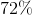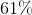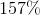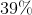Explanation:

The formula for percent error is: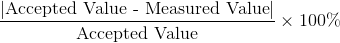In this case, the measured value is 12.8g and the accepted value is 32.9g.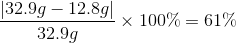### Example Question #1 : Error

A reaction between one mole of sodium and one mole of chloride should yield 42 grams of sodium chloride. In your experiment, the actual yield is 32.73 grams. Calculate the percent error of your experiment.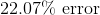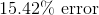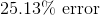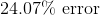Explanation:

To find percent error we need to use the following equation: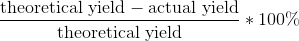Plug in 42 for the theoretical yield and 32.73 for the actual yield and solve accordingly.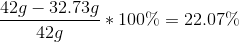### Example Question #1 : Calculating Error

In the following reaction, eight moles of sodium hydroxide is broken down into four moles of sodium oxide and four moles of water. What is the percent error if your experiment yields 195 grams of sodium oxide?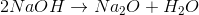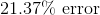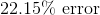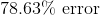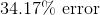Explanation:

To find the percent error we need to use the following equation:But in order to do this, we first have to convert moles of sodium oxide into grams: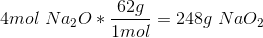This gives us a theoretical yield of 248g, which we plug in with our 195g actual yield.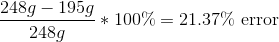### Example Question #1 : Analyzing Data

If a given sample of silver and flourine ideally combine to form 0.6mol of AgF, what is the percent error if the actual yield is 43 grams?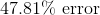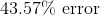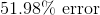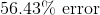Explanation:

Our first step to complete this problem is to convert moles AgF into grams: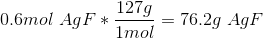This gives us a theoretical yield of 76.2 grams. We can find the percent error by plugging in 76.2g for our theoretical yield and 43g for the actual yield in the following equation: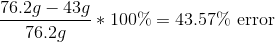### Example Question #62 : High School Chemistry

After conducting an experiment that involved the reaction of solutions of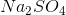and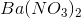, 6.8 grams of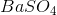was yielded. What is the percent error if the theoretical yield offor this experiment is 7.8 grams?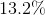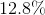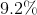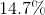Explanation:

Use the following formula to find the percent error: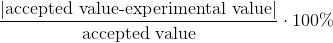For this experiment, our accepted value is the same as the theoretical value.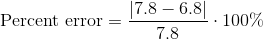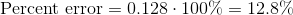### Example Question #1 : Precision, Accuracy, And Error

A student measures that a piece of string is 2.6 cm, but the actual length of the string is 2.9 cm. What is the student's percent error?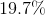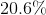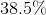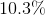Explanation:

Use the following formula to find the percent error:For this experiment, our accepted value is the same as the theoretical value.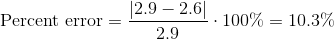### Example Question #1 : Calculating Error

A standard mass of 100.0 grams was placed on a balance. The balance indicated that the mass was 101.6 grams. What is the percent error for the balance?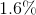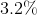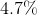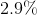Explanation:

Use the following formula to find the percent error:For this experiment, our accepted value is the same as the theoretical value.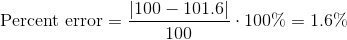### Example Question #1 : Precision, Accuracy, And Error

From an experiment, a student found the density of a rubber eraser was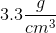. However, the actual density of the eraser is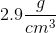. What is the student's percent error?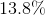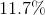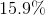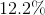Explanation:

Use the following formula to find the percent error:For this experiment, our accepted value is the same as the theoretical value.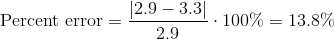### Example Question #1 : Precision, Accuracy, And Error

The experimental value obtained for the specific heat of gold was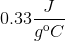. The known specific heat of gold is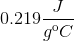. What is the percent error?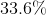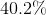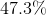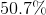Explanation:

Use the following formula to find the percent error:For this experiment, our accepted value is the same as the theoretical value.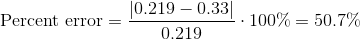### Example Question #6 : Calculating Error

A reaction produced 98.5 grams of nitrogen dioxide gas. However, the calculated theoretical value indicates that 115.2 grams of nitrogen dioxide gas should have been produced. What is the percent error?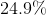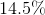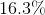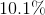Explanation:

Use the following formula to find the percent error:For this experiment, our accepted value is the same as the theoretical value.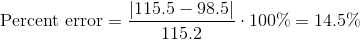← Previous 1

### All High School Chemistry Resources